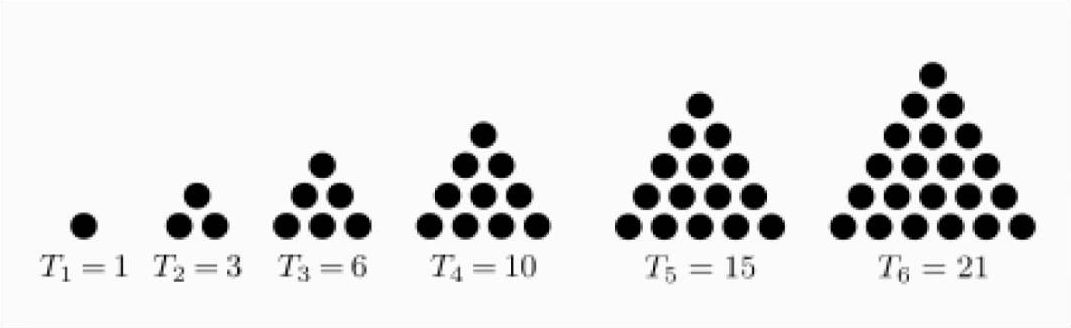# 巴斯卡三角與期望值Pascal 用他的「Arithmetic Triangle」來回答 Problem of Points。 假如賭金是 $$P$$，則「公平的」分配是：其中一人取得 ${R\over 2^n}\times P$ 其中 $$R$$ 是巴斯卡三角第 $$n$$ 列前 $$k$$ 項的和，也就是 ${C^n_0+C^n_1+\cdots+C^n_{k-1}\over 2^n}\times P$ 當然 $$k\leq n$$（k is not greater than n），而另一人取得剩下的賭金。 以上 n 和 k 的意義，請看：

 [語音講解：pascal.mp3]

[ 回上層 ]

Created: Mar 14, 2022
Last Revised: